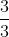# Common Core: 3rd Grade Math : Understand a Fraction: CCSS.Math.Content.3.NF.A.1

## Example Questions

← Previous 1 2 Next →

### Example Question #221 : Fractions

What fraction of the circle is green?Explanation:

The circle is split up intoequal parts.of the parts is shaded green, which meansof the circle is green.

### Example Question #12 : Understanding Fractions As Numbers

What fraction of the circle is green?The circle is split up intoequal parts.of the parts are shaded green, which meansof the circle is green.